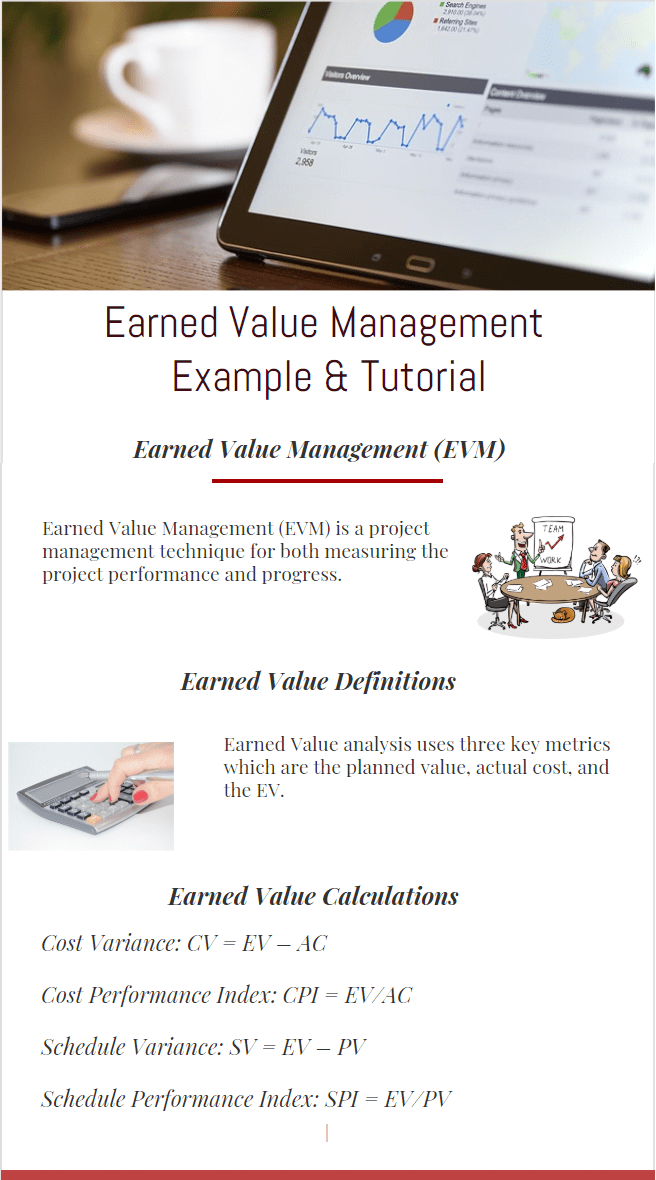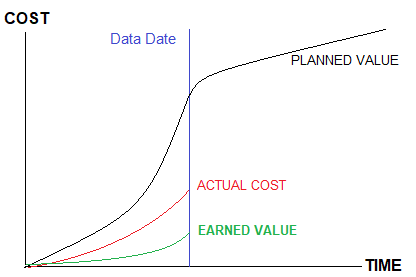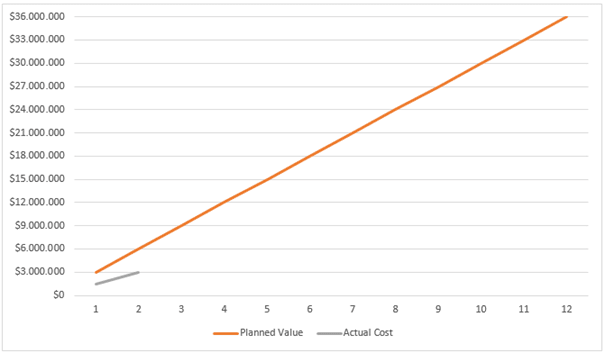## Earned Value Management Example & Tutorial

One week ago, we received an email from one of our readers asking where to find earned value management exercises and answers . In this earned value management tutorial , we will analyze an Earned Value Management Example . Basically, earned value analysis is an efficient technique to realise and manage the project’s performance. Organizations across all the industries use Earned Earned Value analysis techniques to understand the health of their projects. Customers and/or the stakeholders usually ask Project managers these famous questions :

How is the project going ? or How is the performance of the project ?

One traditional technique often used by project managers is to compare the planned and the actual cost curves to answer.

If the actual cost is less than the planned cost, can we say that the projects cost performance is well ? or are we ahead of schedule ?

The planned and the actual curve comparison is not enough to understand how the project is going. This article first reviews the term “Earned Value Management” and formulas. Then, demonstrates the Earned Value calculations by using an example.

### Earned Value Management (EVM)

Common project management methods use the actual vs. planned model to monitor how the project is successfull form a budgeting viewpoint. But this kind of traditional methods do not keep in mind the value of the work performed in a given period. Earned Value Management (EVM) is a project management technique for both measuring the project performance and progress.

While using the actual vs. planned model, only the under or overspending can be reported. It does not show your progress performance. For instance while being ahead of the plan and making enormous progress, overspending could be seen and as a result, the project will finish earlier.

However, if there is no method for understanding the value of work performed, you have no chance to report what the main reasons for the overspend are. Project percent complete values can be monitored from the work schedule but it is not easy to link this percent complete value with actual cost and budget.
For better understanding, let’s analyze a sample project in terms of actual vs. planned and use earned value to understand what are the benefits of this method.

### Earned Value Definitions

Earned Value analysis uses three key metrics which are the planned value, actual cost, and the EV as illustrated in the figure below.PLANNED VALUE ACTUAL COST EV

Planned Value (PV),  is the budgeted cost for the work scheduled or budgeted cost of work scheduled (BCWS).

Actual Costs (AC), actual cost for the work accomplished or actual cost of work performed (ACWP).

Earned Value (EV) is the percent of the total budget actually completed at a point in a given time. It is also known as the budgeted cost of work performed (BCWP)

For example if total scope of work is to install 1000 transmission tower, and 600 of them are installed, the progress of the work is 60% complete. If the total budgeted cost of this work is \$3 M, the EV is \$3 M x 0,6 = \$ 1,8 M.

### Earned Value Calculations

EV Calculation formulas are;

Cost Variance: CV = EV – AC

Cost Performance Index: CPI = EV/AC

Schedule Variance: SV = EV – PV

Schedule Performance Index: SPI = EV/PV

If Cost Performance Index (CPI) is less than one and a negative CV means that the project cost performance is below the plan.

If Schedule Performance Index (SPI) is greater than one and a positive SV means that you completed more work than planned. But don’t forget that SPI > 1 does not always mean that you are ahead of schedule. You should check the critical path and determine if you are behind or ahead of schedule.

## Earned Value Management ExampleEarned Value Management Example – Planned Value,Actual Cost

Let’s assume a 12 month railway project that is planned to spend \$3M per month for a total budget at completion (BAC) of \$36M. The project is 2 months along and according to the planned vs. actual cost figures is underspent by 50%.
We could assume therefore that the project is going well because it’s costing less than planned.

But after 2 months of work, 10 % of work has been completed so “EV” is 10% of the total \$36M budget, which is \$3.6M.

Budget at Completion (BAC) : \$36M
Planned Value (PV) for 2 Months : \$6M
EV : \$3.6M
Actual Cost (AC): \$3M

Schedule Variance (SV) : (Earned Value – Planned Value) = \$3.6 – \$6 = – \$2.4 Behind the schedule
Cost Variance (CV): (Earned Value – Actual Cost) = \$ 600K Under Budget
Cost Performance Index (CPI): (Earned Value / Actual Cost) = 1.2
Schedule Performance Index (SPI): (Earned value / Planned value) = 0.6
Estimate at Completion (EAC) : (Budget at Completion / Cost Performance Index) =\$30M

Estimate to Completion (ETC): (Budget at Completion – Earned Value)/ Cost Performance Index = \$27 M

Time to Complete: (Budget at Completion – EV)/ Schedule Performance Index =54 Months

By taking into consideration of this performance, this railway project will be completed in 54 months with a \$36 M budget.

### Summary

Earned value is a powerful technique which provides project information by the help of project schedule, planned value, actual value and earned value calculations. It is widely used by stakeholders to understand the real performance of the project.

This Earned Value Management tutorial we analyzed an Earned Value Management Example which emphasizes the benefits of this system in project management. At the beginning and the end of a project, because of the lack of coordination between crews and equipment, low performance will occur. It is better to be aware of delays in the early stages by using project management tools to ensure project success.

Tags:
or